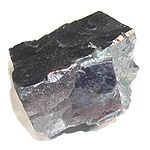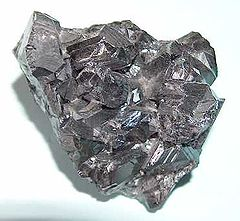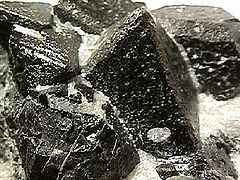# Heat Capacities of Minerals

$$\newcommand{\vecs}{\overset { \rightharpoonup} {\mathbf{#1}} }$$ $$\newcommand{\vecd}{\overset{-\!-\!\rightharpoonup}{\vphantom{a}\smash {#1}}}$$$$\newcommand{\id}{\mathrm{id}}$$ $$\newcommand{\Span}{\mathrm{span}}$$ $$\newcommand{\kernel}{\mathrm{null}\,}$$ $$\newcommand{\range}{\mathrm{range}\,}$$ $$\newcommand{\RealPart}{\mathrm{Re}}$$ $$\newcommand{\ImaginaryPart}{\mathrm{Im}}$$ $$\newcommand{\Argument}{\mathrm{Arg}}$$ $$\newcommand{\norm}{\| #1 \|}$$ $$\newcommand{\inner}{\langle #1, #2 \rangle}$$ $$\newcommand{\Span}{\mathrm{span}}$$ $$\newcommand{\id}{\mathrm{id}}$$ $$\newcommand{\Span}{\mathrm{span}}$$ $$\newcommand{\kernel}{\mathrm{null}\,}$$ $$\newcommand{\range}{\mathrm{range}\,}$$ $$\newcommand{\RealPart}{\mathrm{Re}}$$ $$\newcommand{\ImaginaryPart}{\mathrm{Im}}$$ $$\newcommand{\Argument}{\mathrm{Arg}}$$ $$\newcommand{\norm}{\| #1 \|}$$ $$\newcommand{\inner}{\langle #1, #2 \rangle}$$ $$\newcommand{\Span}{\mathrm{span}}$$$$\newcommand{\AA}{\unicode[.8,0]{x212B}}$$

The amount of heat energy required to cause a temperature change of 100 °C in 1 m3 of quartz is not the same as the amount of heat energy required for a 100 °C change in 1 m3 of magnetite. The quartz block requires only 195 MJ, while the magnetite requires 320 MJ. Conversely, If 100 MJ of heat is added to each block, the temperature of the quartz rises by about 52 °C, while the magnetite will rise by only 21.5 °C.

The difference is due to the differing heat capacities of quartz and magnetite. The heat capacity of minerals is one of the critical physical properties used to evaluate the proposed Yucca Mountain spent nuclear fuel repository , or to understand heat flow in the Earth in general. The relationship between heat and temperature is important in petrology (the study of rocks and the conditions under which they form). Understanding heat flow and temperature is a logical entry point to geological thermodynamics. A USGS publication  provides extensive geothermal data like the following:

Table $$\PageIndex{1}$$ Specific heat capacities (25 °C unless otherwise noted)

Mineral Photo Formula Heat Capacity
(J/mol°C)
Kopp Value Density
galenaPbS 49.5 ~7.4
sphalerite (zincblende)ZnS
(Zn,Fe)S
46.1 ~4.0
pyriteFeS2 62.2 ~5.0
quartzSiO2 44.5 48.9 ~2.6
corundumAl2O3 79.1 ~4.0
magnetiteFe3O4 143.5 ~5.17

## Specific Heat Capacities

When we supply heat energy to a mineral, a rise in temperature occurs (provided that no chemical changes or phase changes take place). The rise in temperature is proportional to the quantity of heat energy supplied. If q is the quantity of heat supplied and the temperature rises from T1 to T2 then

$\text{q} = \text{C} × \text{(T}_2 – \text{T}_1)$

OR

$\text{q} = \text{C} × (\Delta \text{T})$

where the constant of proportionality C is called the heat capacity of the sample. The sign of q in this case is + because the sample has absorbed heat (the change was endothermic), and (ΔT) is defined in the conventional way.

If we add heat to any homogenous sample of matter of variable mass, such as a pure substance or a solution, the quantity of heat needed to raise its temperature is proportional to the mass as well as to the rise in temperature. That is,

$\text{q} = \text{C} × \text{m} × (\text{T}_2–\text{T}_1)$

OR

$\text{q} = \text{C} × \text{m} × (\Delta \text{T})$

The new proportionality constant C is the heat capacity per unit mass. It is called the specific heat capacity (or sometimes the specific heat), where the word specific means “per unit mass.”

Specific heat capacities provide a convenient way of determining the heat added to, or removed from, material by measuring its mass and temperature change. As mentioned [|previously], James Joule established the connection between heat energy and the intensive property temperature, by measuring the temperature change in water caused by the energy released by a falling mass. In an ideal experiment, a 1.00 kg mass falling 10.0 m would release 98.0 J of energy. If the mass drove a propeller immersed in 0.100 liter (100 g) of water in an insulated container, its temperature would rise by 0.234oC. This allows us to calculate the specific heat capacity of water:

$$\text{98 J} = \text{C} × \text{100 g} × \text{0.234} ^\circ C$$
$$\text{C} = \text{4.184} \dfrac{J}{g ^\circ C }$$

At 15°C, the precise value for the specific heat of water is 4.184 J K–1 g–1, and at other temperatures it varies from 4.178 to 4.218 J K–1 g–1. Note that the specific heat has units of g (not the base unit kg), and that since the Centigrade and kelvin scales have identical graduations, either oC or K may be used.

## Specific Heat Capacities of Minerals

The specific heat capacities of minerals can be estimated by adding the heat capacities of the constituent atoms. This method accentuates the fact that the heat capacity depends on the number of atoms present in the sample. The heat capacity of quartz is thus calculated from values in the table below:

CV (silicon) + 2 x CV (oxygen) = CV (quartz) ~ CP (quartz)

15.9 + 2 x 16.7 = 48.9 J / mol °C

Table $$\PageIndex{2}$$: Kopp Values of CV

Atom Heat Capacity
(J/mol°C)
H 10.5
Be 12.5
B 10.5
C 8.4
N 12.5
O 16.7
F 20.9
Al 14.5
Si 15.9
P 22.7
S 22.7
All heavier
elements
25.9

*The Kopp values are for heat capacities measured at constant volume and denoted CV; other heat capacities on this page are measured at constant pressure and denoted CP, but CV is usually very close to CP.

Example $$\PageIndex{1}$$: Heat Capacity of Fluorite

Calculate the heat capacity of Fluorite, CaF2, and determine how much heat is required to raise the temperature of 0.5 m3 of fluorite (D = 3.2) from 25.0 oC to 125 oC. Assume that CP = CV

Solution: From the Table,

$$\text{C}_V (\text{CaF}_2) = 25.9 + 2 * 20.9 = \text{67.7} \dfrac{J}{mol ^\circ C}$$

$$\text{M} = \text{78.07} \dfrac{g}{mol}$$

$$\text{m} = \text{D} * \text{V} = \text{3.2} \dfrac{g} {cm^3} * (\text{10}^6 \dfrac{cm^3} {m^3}) * \text{0.5} \text{m^3} = \text{1.6 x 10}^6 \text{g}$$

$$\text{q} = \text{C} * \text{m} * (\Delta \text{T})$$

$$\text{C} = \dfrac{67.7 \dfrac{J}{mol^\circ C}} {78.07 g/mol} = \text{0.867} \dfrac{ J} {g^\circ C}$$

$$\text{q} = \text{0.867} \dfrac{J}{g^\circ C} × \text{1.6 x 10}^6 g × \text{(125.0 - 25.0)}^\circ C = \text{1.39 x 101.6 x 10}^8 J$$
$$\text{q} = \text{139 MJ}$$

Table $$\PageIndex{3}$$ Specific Heat Capacities (25 °C unless otherwise noted)

Substance phase Cp(see below)
J/(g·K)
air, (Sea level, dry, 0 °C) gas 1.0035
argon gas 0.5203
carbon dioxide gas 0.839
helium gas 5.19
hydrogen gas 14.30
methane gas 2.191
neon gas 1.0301
oxygen gas 0.918
water at 100 °C (steam) gas 2.080
water at 100 °C liquid 4.184
ethanol liquid 2.44
water at -10 °C (ice)) solid 2.05
copper solid 0.385
gold solid 0.129
iron solid 0.450

## Electrical Energy Conversion

The most convenient way to supply a known quantity of heat energy to a sample is to use an electrical coil. The heat supplied is the product of the applied potential V, the current I flowing through the coil, and the time t during which the current flows:

$\text{q} = \text{V} × \text{I} × \text{t}$

If the SI units volt for applied potential, ampere for current, and second time are used, the energy is obtained in joules. This is because the volt is defined as one joule per ampere per second:

$$\text{1 volt} × \text{1 ampere} × \text{1 second} = \text{1} \dfrac{J}{A s} × \text{1 A} × \text{1 s} = \text{1 J}$$

Example $$\PageIndex{2}$$: Heat Energy

An electrical heating coil, 230 cm3 of water, and a thermometer are all placed in a polystyrene coffee cup. A potential difference of 6.23 V is applied to the coil, producing a current of 0.482 A which is allowed to pass for 483 s. If the temperature rises by 1.53 K, find the heat capacity of the contents of the coffee cup. Assume that the polystyrene cup is such a good insulator that no heat energy is lost from it.

Solution The heat energy supplied by the heating coil is given by

$$\text{q} = \text{V} × \text{I} × \text{t} = \text{6.23 V} × \text{0.482 A } × \text{483 s} = \text{1450 V A s} = \text{1450 J}$$

However,

$$\text{q} = \text{C} × \text{(T}_2 – \text{T}_1)$$

Since the temperatue rises, T2 > T1 and the temperature change ΔT is positive:

$$\text{1450 J} = \text{C} × \text{1.53 K}$$

so that

$$\text{C} = \dfrac{1450 J}{1.53 K} = \text{948} \dfrac{J}{K}$$

Note

Note: The heat capacity found applies to the complete contents of the cup-water, coil, and thermometer taken together, not just the water.

As discussed in other sections, an older, non-SI energy unit, the calorie, was defined as the heat energy required to raise the temperature of 1 g H2O from 14.5 to 15.5°C. Thus at 15°C the specific heat capacity of water is 1.00 cal K–1 g–1. This value is accurate to three significant figures between about 4 and 90°C.

If the sample of matter we are heating is a pure substance, then the quantity of heat needed to raise its temperature is proportional to the amount of substance. The heat capacity per unit amount of substance is called the molar heat capacity, symbol Cm. Thus the quantity of heat needed to raise the temperature of an amount of substance n from T1 to T2 is given by

$\text{q} = \text{C} × \text{n} × (\text{T}_2 – \text{T}_1)\label{6}$

The molar heat capacity is usually given a subscript to indicate whether the substance has been heated at constant pressure (Cp)or in a closed container at constant volume (CV).

Example $$\PageIndex{3}$$: Heat Supply

A sample of neon gas (0.854 mol) is heated in a closed container by means of an electrical heating coil. A potential of 5.26 V was applied to the coil causing a current of 0.336 A to pass for 30.0 s. The temperature of the gas was found to rise by 4.98 K. Find the molar heat capacity of the neon gas, assuming no heat losses.

Solution The heat supplied by the heating coil is given by

$$\text{q} = \text{V} × \text{I} × \text{t}$$
= $$\text{5.26 V} × \text{0.336 A} × \text{30.0 s}$$
= $$\text{53.0 V A s}$$
= $$\text{53.0 J}$$

Rearranging Eq. $$\ref{6}$$, we then have

$$\text(C)_m = \dfrac{q}{n(T_2-T_1)} = \dfrac{53.0 J}{0.854 mol * 4.98 K} = \text{12.47} \dfrac{J}{mol*k}$$

However, since the process occurs at constant volume, we should write

$$\text{C}_V = \text{12.47} \dfrac{J}{mol*K}$$

From ChemPRIME: 15.1: Heat Capacities

## References

1. http://www.osti.gov/bridge/purl.cover.jsp;jsessionid=CD3A8DE617358EBB32CF145C0AF640DD?purl=/838658-hkUqgt/native/
2. http://en.Wikipedia.org/wiki/Galena
3. en.Wikipedia.org/wiki/Sphalerite
4. en.Wikipedia.org/wiki/Pyrite
5. en.Wikipedia.org/wiki/Quartz
6. en.Wikipedia.org/wiki/Corundum
7. en.Wikipedia.org/wiki/Magnetite
8. Bailey, D.G. Heat Capacity of Minerals: A hands-On Introduction to Chemical Thermodynamics", in Brady, J.B; Mogk, D.W.; Perkins II, D. Teaching Mineralogy, Mineralogical Society of America, 1997.